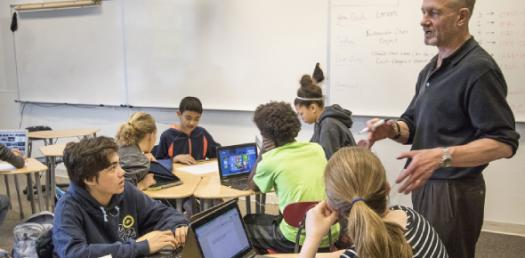# 6th Grade Social Studies Chapter 2

17 Questions | Total Attempts: 495SettingsA map is a very important tool and can show countries and continents we have never been to. Having just finished the second chapter in the class today you are expected to be able to read a map correctly and read directions. Take the test below to see what you understood about maps today.

• 1.
A ___________  is a landform with high elevation and a more or less level surface.
• A.

Plateau

• B.

Mountain

• C.

Hill

• D.

Plains

• 2.
Mapmakers use a framework of lines, called a ___________________, to represent Earth's curved surface on a flat map
• A.

Topographic

• B.

Prime meridian

• C.

Hemisphere

• D.

Map projections

• 3.
The ______- of the North Pole is 90 degrees north.
• A.

Longitude

• B.

Latitude

• C.

Equator

• D.

Prime meridian

• 4.
A spherical model of Earth's entire surface is called a _____________
• A.

Crust

• B.

Map

• C.

Globe

• D.

Equator

• 5.
Lines of longitude converge at Earth's _____________
• A.

Relief

• B.

Sattelite images

• C.

Prime meridian

• D.

Great plains

• 6.
Pictures of Earth's surface, called ______________, are based on data obtained by Landsat satellites.
• A.

Hemisphere

• B.

Stratosphere

• C.

Satellite images

• D.

Television

• 7.
Computer mapmakers use the process of ___________ to convert the location of points on a map to numbers
• A.

GPS

• B.

Digitizing

• C.

Scale

• D.

Topographic

• 8.
The _______________ is a satellite-based system that can precisely determine latitude and longitude for points on Earth's surface
• A.

GPS

• B.

Landforms

• C.

Latitude

• D.

Symbols

• 9.
A _________ map uses coutour lines to show the elevation, relief, and slope of an area
• A.

Latitude

• B.

Longitude

• C.

Topographic

• D.

Elevation

• 10.
The __________ is the difference in elevation between two contour lines that are side by side
• A.

Relief

• B.

Countour interval

• C.

Contour lines

• D.

Prime meridian

• 11.
In the United States, many topographic maps have a ______________ of 1 : 24,000
• A.

Relief

• B.

Scale

• C.

Symbols

• D.

Gps

• 12.
Roads, rivers, airports, and woods are represented by different _____________ on a topograhphic map.
• A.

Scale

• B.

Line

• C.

Symbol

• D.

Data

• 13.
On a topographic map, points of equal elevation are connected by ______________
• A.

Lateral lines

• B.

Crust

• C.

Hemispheres

• D.

Contour lines

• 14.
Each dot, or ____________- in a satellite image contains information abouth the color and brightness of a small area on Earth's surface
• A.

• B.

Pixel

• C.

Image

• D.

Relief

• 15.
A world map drawn using a __________ projection would correctly show the relative sizes of Earth's landmasses.
• A.

Map

• B.

Equal area

• C.

Unequal area

• D.

Latitude

• 16.
A large area of land where the topography is similar is called a ____________ region
• A.

Mountain

• B.

Plateau

• C.

Plains

• D.

Landform

• 17.
One thing that the equator and prime meridian have in common is that they divide the globe into ________________
• A.

Prime meridian

• B.

Hemispheres

• C.

Latitudes

• D.

Longitude

Related TopicsBack to top